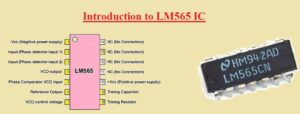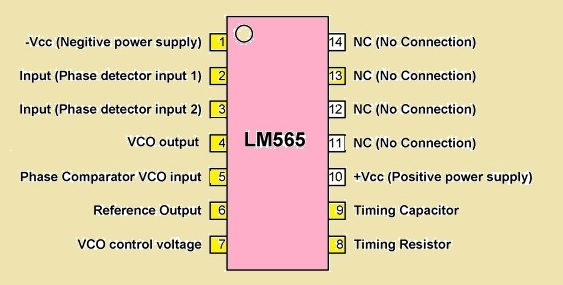Hello, readers welcome to new post. Today we will learn Introduction to LM565 IC. This integrated circuit is created for different applications such as division of frequency multiplication of frequency and demodulation and is known as phase-locked loop IC. It has 2 main parts first one is a voltage-controlled oscillator and the second one is a phase detector.

This post will cover its basics pinouts features working and some other paramerts. So let get started Introduction to LM565 IC.

### Introduction to LM565 IC

• The LM565 phase-locked IC used in general purpose applications and comprises of linear voltage controller oscillator and a stable circuit that helps for less distorted modulation. It also comes with a double-phase detector system that offers high-quality carrier suppression.
•  For  adjustment, the frequency of VCO exterior capacitor and resistance is used and a tuning range of ratio 10:1 can get with the use of a connected capacitor
• Its different features such as closed-loop system, bandwidth, and capture response speed can be regulated through use of resistor and capacitor
• Its loop can be damaged among the VCO and phase detector for placement of a digital frequency divider to get eh frequency multiplication
• Its operating temperatures range is minus fifty-five to one twenty-five centigrade.### Features of LM565 IC

• Its main features are discussed here
• Its highest power dissipation is forteen hundred megawatt
• Its power supply current value is 12.5 mA
• The value of storage temperature is minus sixty-five to one fifty centigrade
• The highest operating temperatures is plus-minus five volts to plus-minus twelve volts
• The operating frequency for VCo is five hundred kilohertz
• It can operate t different values of input volts
• It gives the 0.2 percent linearity for demodulated output

### Pinout of LM565 IC

• Its main pinout is explained here
• Pin 1 -Vcc that used for negative power supply
• Pin 2 is used for phase detector
• Pin 3 is also a phase detector input
• Pin 4 VCO output pin
• Pin 5 is input of the phase detector
• Pin 6 is the inner amplifier reference o/p
• Pin 7 used for VCO volts control
• Pin 8 is resistance for the free-running frequency of VCO
• Pin 9 Timing capacitor for VCO
• Pin +Vcc Here positive supply given
• Pin 11 to 14 are not in use### LM565 IC Applications

• Common applications of LM565 are explained here
• it used in coherent demodulators
• Used for generation of signal